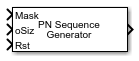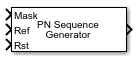# PN Sequence Generator

Generate pseudonoise sequence

• Library:
• Communications Toolbox / Comm Sources / Sequence Generators

Communications Toolbox HDL Support / Comm Sources

•## Description

The PN Sequence Generator block generates a sequence of pseudorandom binary numbers using a linear-feedback shift register (LFSR). Pseudonoise sequences are typically used for pseudorandom scrambling, and in direct-sequence spread-spectrum systems. For more information, see More About.

These icons shows the block with all ports enabled.## Ports

### Input

expand all

Output mask to delay the PN sequence from initial time, specified as a binary vector with N elements. N is the degree of the generator polynomial.

#### Dependencies

To enable this port, set Output mask source to `Input port`.

Data Types: `double` | `uint8` | `ufix1`

Output size for variable-size output signals, specified as an integer. For information about variable-size signals, see Variable-Size Signal Basics (Simulink).

#### Dependencies

To enable this port, select Output variable-size signals and set Maximum output size source to ```Dialog parameter```.

Data Types: `double`

Reference input, specified as a column vector that determines the maximum and current output sequence length. The Ref input must be a variable-size signal. For information about variable-size signals, see Variable-Size Signal Basics (Simulink).

#### Dependencies

To enable this port, select Output variable-size signals and set Maximum output size source to ```Inherit from reference input```.

Data Types: `double`

Reset sequence generator, specified as `0` or `1`. For more information, see Reset Behavior.

#### Dependencies

To enable this port, select Reset on nonzero input.

Data Types: `Boolean`

### Output

expand all

PN sequence, returned as a binary vector.

## Parameters

expand all

Generator polynomial that determines the feedback connections of the shift register, specified as one of these options:

• Character vector or string scalar of a polynomial whose constant term is `1`. For more information, see Representation of Polynomials in Communications Toolbox.

• Binary-valued row vector that represents the coefficients of the polynomial in order of descending powers. The length of this vector must be N + 1, where N is the degree of the polynomial. The first and last entries must be `1`, indicating the leading term with degree N and a constant term of 1.

• Integer-valued row vector of elements that represent the exponents for the nonzero terms of the polynomial in order of descending powers. The last entry must be `0`, indicating a constant term of 1.

Example: `'z^8 + z^2 + 1'`, `[1 0 0 0 0 0 1 0 1]`, and `[8 2 0]` represent the same polynomial, p(z) = z 8 + z 2 + 1.

Data Types: `double` | `char`

Initial shift register states of the PN sequence generator when the simulation starts, specified as a binary-valued row vector. The length of the vector must equal the degree of the generator polynomial specified by the Generator polynomial. For more information, see Simple Shift Register Generator.

Note

For the block to generate a nonzero sequence, the Initial states vector must contain at least one nonzero element.

Data Types: `double`

Output mask source that indicates how the output mask information is given to the block, specified as one of these:

• `Dialog parameter` to use the ```Output mask vector (or scalar shift value)``` parameter setting.

• `Input port` to add and use the `Mask` input port.

Output mask vector or scalar shift value, specified as an integer scalar or binary row vector of length N, where N is the degree of the generator polynomial. This parameter determines the delay of the PN sequence from the initial time. For more information, see Shifting PN Sequence Starting Point.

#### Dependencies

To enable this parameter, set Output mask source to `Dialog parameter`.

Data Types: `double`

Select this parameter to enable variable-length output sequences during simulation. When you clear this parameter, the block outputs fixed-length sequences. When you select this parameter, the block can output variable-length sequences. For information about variable-size signals, see Variable-Size Signal Basics (Simulink).

Select how to specify the maximum sequence output size.

• `Dialog parameter` — Select this value to configure the block to use the Maximum output size parameter setting as the maximum permitted output sequence length. The oSiz input port specifies the current size of the output signal, and the block output inherits the sample time from the input signal. The input value of oSiz must be less than or equal to the Maximum output size parameter.

• `Inherit from reference port` — Select this value to enable the Ref input port and configure the block to inherit the sample time, maximum size, and current output size from the variable-sized signal at the Ref input port. These set the maximum permitted output sequence length.

#### Dependencies

To enable this parameter, select Output variable-size signals.

Specify the maximum output size for the block. n is a positive scalar.

Example: `[10 1]` specifies a 10-by-1 maximum size for the output signal.

#### Dependencies

To enable this parameter, and set Maximum output size source to `Dialog parameter`.

Data Types: `double`

Positive scalars specify the time in seconds between each sample of the output signal. If you set the Sample time to `-1`, the output signal inherits the sample time from downstream. For information on the relationship between the Sample time and Samples per frame parameters, see Sample Timing.

Example: `1` specifies a sample time of 1 second.

#### Dependencies

To enable this parameter, clear Output variable-size signals.

Data Types: `double`

Samples per frame in one channel of the output signal, specified as a positive integer. For information on the relationship between Sample time and Samples per frame, see Sample Timing.

#### Dependencies

To enable this parameter, clear Output variable-size signals.

Data Types: `double`

Select this parameter to add the `Rst` input port. For more information, see Reset Behavior.

Select this parameter to make the Number of packed bits and Interpret bit-packed values as signed parameters available.

When this parameter is selected, the object outputs a column vector of length M, which contains most-significant-bit (MSB) first integer representations of bit words of length P. M is the number of samples per frame specified in the Samples per frame parameter. P is the size of the bit-packed words specified in the Number of packed bits parameter.

Note

The first bit from the left in the bit-packed word contains the most significant bit for the integer representation.

Number of packed bits, specified as an integer in the range [1, 32].

#### Dependencies

To enable this parameter, select Enable bit-packed outputs.

Data Types: `double`

Interpret bit-packed values as signed integer data values when selected or unsigned integer data values when cleared. When selected, a 1 in the most significant bit (sign bit) indicates a negative value.

#### Dependencies

To enable this parameter, select Enable bit-packed outputs.

Output data type, specified as `double`, `boolean`, or ```Smallest unsigned integer```.

• When Enable bit-packed outputs is cleared, the output data type can be specified as a `double`, `boolean`, or `Smallest unsigned integer`. When the Output data type parameter is set to `Smallest unsigned integer`, the output data type is selected based on the settings used in the Hardware Implementation pane of the Configuration Parameters dialog box of the model. If `ASIC/FPGA` is selected in the Hardware Implementation pane, the output data type `ufix(1)` = ideal minimum one-bit size. For all other selections, it is an unsigned integer with the smallest available word length large enough to fit one bit, usually corresponding to the size of a char (for example, `uint8`).

• When Enable bit-packed outputs is selected, the output data type can be specified as `double` or ```Smallest unsigned integer```. When the Output data type parameter is set to `Smallest unsigned integer`, the output data type is selected based on the Interpret bit-packed values as signed and Number of packed bits parameters, and the settings used in the Hardware Implementation pane of the Configuration Parameters dialog box of the model. If `ASIC/FPGA` is selected in the Hardware Implementation pane, the output data type is the ideal minimum n-bit size, such as `sfix(n)` or `ufix(n)`, based on the Interpret bit-packed values as signed parameter. For all other selections, it is a signed or unsigned integer with the smallest available word length large enough to fit n bits.

## Block Characteristics

 Data Types `Boolean` | `double` | `fixed point` Multidimensional Signals `no` Variable-Size Signals `yes`

expand all

## Compatibility Considerations

expand all

Behavior changed in R2020a

 Proakis, John G. Digital Communications. 3rd ed. New York: McGraw Hill, 1995.

 Lee, J. S., and L. E. Miller. CDMA Systems Engineering Handbook. Boston and London. Artech House, 1998.

 Golomb, S.W. Shift Register Sequences. Laguna Hills. Aegean Park Press, 1967.